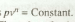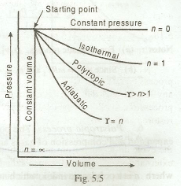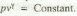General Laws for Expansion and Compression

The general law of expansion or compression of a perfect gas is:It gives the relationship between pressure and volume of a given quantity of gas. The value of n depends upon the nature of gas, and condition under which the changes (i.e. expansion or compression) takes place. The value of n may be between zero and infinity. But the following values of n are important from the subject point of view:1. When n = 0, then pv° = Constant, i.e. p = Constant. In other words, for the expansion or compression of a perfect gas at constant pressure, n= 0.

2. When n = 1, then pv = Constant, i.e. the expansion or compression is isothermal or hyperbolic.

3. When n lies between 1 and n, the expansion or compression is polytropic, i.e.4. When n = Ɣ, the expansion or compression is adiabatic, i.e.5. When n = ∞, the expansion or compression is at constant volume, i.e. v = Constant.

Fig 5.5 shows the curves of expansion of a perfect gas for different values of n. We see that greater the value of n, steeper is the curve of expansion.